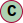# Class Absolute3DPosition

Absolute cartesian 3D position. This class uses a Vector3. This location can be used both as an absolute location or relative location.

#### Hierarchy-Diagram##### Legendclass

## Properties

orientation: Orientation

Orientation at recorded position

referenceSpaceUID: string

Position reference space UID

timestamp: number = ...

Position recording timestamp

unit: LengthUnit = LengthUnit.METER

Position unit

vector: Vector3 = ...
velocity: Velocity = ...

Velocity at recorded position

## Accessors

• get accuracy(): Accuracy<LengthUnit, any>
• Position accuracy

#### Returns Accuracy<LengthUnit, any>

Position accuracy

• set accuracy(value): void
• Position accuracy

#### Returns void

• get angularVelocity(): AngularVelocity
• Get the angular velocity

#### Returns AngularVelocity

Angular velocity

• set angularVelocity(value): void
• Set the angular velocity

#### Returns void

• get linearVelocity(): LinearVelocity
• Get the linear velocity

#### Returns LinearVelocity

Linear velocity

• set linearVelocity(value): void
• Set the linear velocity

#### Returns void

• get probability(): number
• Get the position probability

#### Returns number

Probability between 0 and 1

• set probability(value): void
• Position probability

## Methods

• Get the angle in radians from this position to a destination

#### Parameters

• ##### destination: Absolute2DPosition

Destination position

#### Returns number

Bearing in radians from this position to destination

• Get the distance from this location to a destination

#### Parameters

• ##### destination: Absolute3DPosition

Destination location

• ##### `Optional`distanceFunction: DistanceFn = EUCLIDEAN

Distance function to use (default EUCLIDEAN distance)

#### Returns number

Distance between this point and destination

• Set the accuracy of the absolute position

#### Parameters

• ##### accuracy: number | Accuracy<LengthUnit, any>

Accuracy object or number

• ##### `Optional`unit: Unit

Optional unit

instance